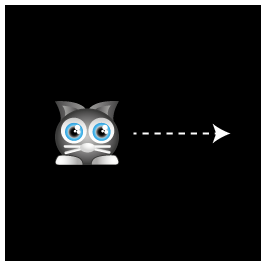## 移动精灵

function setup() {  //Start the game loop by adding the gameLoop function to  //Pixi's ticker and providing it with a delta argument.  app.ticker.add(delta => gameLoop(delta));}function gameLoop(delta){  //Move the cat 1 pixel  cat.x += 1;}cat.x += 1;

delta的值代表帧的部分的延迟。你可以把它添加到cat的位置，让cat的速度和帧率无关。下面是代码:

cat.x += 1 + delta;

function gameLoop() {  //Call this gameLoop function on the next screen refresh  //(which happens 60 times per second)  requestAnimationFrame(gameLoop);  //Move the cat  cat.x += 1;}//Start the loopgameLoop();

//Aliaseslet Application = PIXI.Application,    Container = PIXI.Container,    loader = PIXI.loader,    resources = PIXI.loader.resources,    TextureCache = PIXI.utils.TextureCache,    Sprite = PIXI.Sprite,    Rectangle = PIXI.Rectangle;//Create a Pixi Applicationlet app = new Application({    width: 256,    height: 256,                           antialias: true,    transparent: false,    resolution: 1  });//Add the canvas that Pixi automatically created for you to the HTML documentdocument.body.appendChild(app.view);loader  .add("images/cat.png")  .load(setup);//Define any variables that are used in more than one functionlet cat;function setup() {  //Create the cat sprite  cat = new Sprite(resources["images/cat.png"].texture);  cat.y = 96;  app.stage.addChild(cat);  //Start the game loop  app.ticker.add(delta => gameLoop(delta));}function gameLoop(delta){  //Move the cat 1 pixel  cat.x += 1;  //Optionally use the delta value  //cat.x += 1 + delta;}

（注意 cat 变量需要在setupgameLoop函数之外定义，然后你可以在全局中任何地方都能获取到它们）